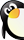### 四年级数学下册 3 运算定律与简便计算（第4课时）乘法分配率习题 新人教版• 需消耗0金币
• 0个贡献点
• 0个黄金点

• 资源类别 试题
资源子类 一课一练
• 教材版本 不限
所属学科 小学数学
• 适用年级 四年级
适用地区 全国通用
• 文件大小 624 K
上传用户 conan01
• 更新时间 2016/5/25 10:15:34
下载统计 今日0 总计47
• 评论(0) 发表评论 报错(0) 我要报错 收藏
1
0

（28＋25）×4=□×4+□×4　9×(7+6)=9×□+□×□
(33+25) ×2= □×□+□×□　15×24+12×15=□×(□+□)
(32+47)×9=32×□+□×9　6×47+6×53=□×(□+□)
(13+□)×10=□×10+7×□　3×7+7×7=□×(□+□)

108×75        101×83      99×83          7×75－7×25
88×27＋27×12        87×53＋87×47        150×45－45×50
、应用题
1．一件毛衣95元，一件呢大衣325元．现在各买4件．买毛衣和呢大衣工共用多元？（用两种方法解答）
2． 一个服装店一天卖出70件运动服，上午卖出20件，每件运动服78元．照这样计算，下午比上午卖多少元？（用不同方法解答）

• 暂时没有相关评论